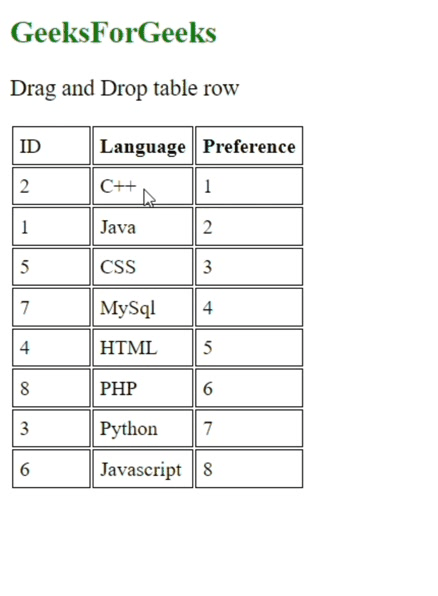Open in App
Not now

# How to create Dynamic Drag and Drop table rows using jQuery Ajax ?

• Difficulty Level : Expert
• Last Updated : 28 Feb, 2023

In this article, we will discuss the method of How to create Dynamic and Drop table rows using JQuery Ajax.  We will use jQuery and HTML CSS to perform the operations of Drag and Drop on the table row.

First to perform the operations of the Drag and Drop table row we need two jQuery libraries without these libraries we can’t perform the Drag and Drop operations on the table row. The required two libraries are:

• jQuery Ajax min.js: It will help us to perform the Drag and Drop operation on the table row:

• jQuery-UI: This as the name suggests, it’s used for showing user interface operations:

Basically Drag and Drop operations on table rows means we can interchange the table row with each other by only operating Drag and Drop operations we need to only Drag and one row Drop it on another row with which we want to perform Drag and Drop operations it’s basically used for change data and for the correctness of data when we write or add wrong data on a table.

Approach: First we create table by using table and td , tr tag in our html code and we add one h1 tag for heading through which i explained what we are making and in first tag or tr we add three column first for id second, language and last one for preference and we add id 1 to 8 and in language we write some coding  language and at last we are adding preference which we will set stable it means when we will perform Drag and Drop operations so that preference will not change their sequences its always static 1 to 8 only two column / row change id and preference according to perform operation by user and after writing code for table we add jQuery libraries without which we can’t operate Drag and Drop operations and the we give id in table GFG and at last we write js first we create one function in which we will use GFG id and we will use sortable method and we use droponEmpty as a false default and after we start operation by using one more function and we addclass select and for stop the operation we will make one other function and set the stop function and write code for stop and successful showing interchange table operations on table row and we set GFG greater than 0 which and use findmethod in which we use td for Drag and Drop operations so with the help of these code we can perform Drag and Drop operations on table row.

Example: This is the implementation of the above approach:

## HTML

 `` `<``html` `lang``=``"en"``>`   `<``head``>` `    ``<``meta` `charset``=``"UTF-8"``>` `    ``<``meta` `http-equiv``=``"X-UA-Compatible"` `          ``content``=``"IE=edge"``>` `    ``<``meta` `name``=``"viewport"` `          ``content``=``"width=device-width, initial-scale=1.0"``>` `    ``<``title``>Document` `` `<``style``>` `    ``table th,` `    ``table td {` `        ``width: 50px;` `        ``padding: 5px;` `        ``border: 0.5px solid black;`     `    ``}`   `    ``.GFG {` `        ``color: green;` `    ``}`   `    ``.OK {` `        ``font-size: 18px;` `    ``}` ``   `<``body``>` `    ``<``h2` `class``=``"GFG"``>GeeksForGeeks` `    ``<``p` `class``=``"OK"``>Drag and Drop table row` `    ``<``table` `id``=``"GFG"``>` `        ``<``tr``>` `            ``<``td``>ID` `            ``<``th``>Language` `            ``<``th``>Preference` `        ``` `        ``<``tr``>` `            ``<``td``>1` `            ``<``td``>Java` `            ``<``td``>1` `        ``` `        ``<``tr``>` `            ``<``td``>2` `            ``<``td``>C++` `            ``<``td``>2` `        ``` `        ``<``tr``>` `            ``<``td``>3` `            ``<``td``>Python` `            ``<``td``>3` `        ``` `        ``<``tr``>` `            ``<``td``>4` `            ``<``td``>HTML` `            ``<``td``>4` `        ``` `        ``<``tr``>` `            ``<``td``>5` `            ``<``td``>CSS` `            ``<``td``>5` `        ``` `        ``<``tr``>` `            ``<``td``>6` `            ``<``td``>Javascript` `            ``<``td``>6` `        ``` `        ``<``tr``>`   `            ``<``td``>7` `            ``<``td``>MySql` `            ``<``td``>7` `        ``` `        ``<``tr``>` `            ``<``td``>8` `            ``<``td``>PHP` `            ``<``td``>8` `        ```   `    ``` `    ``<``script` `type``=``"text/javascript"` `src``=` `"https://ajax.googleapis.com/ajax/libs/jquery/1.8.3/jquery.min.js"``>` `      ``` `    ``<``link` `rel``=``"stylesheet"` `        ``href``=` `"https://ajax.googleapis.com/ajax/libs/jqueryui/1.8.24/themes/smoothness/jquery-ui.css"` `/>` `    ``<``script` `type``=``"text/javascript"` `        ``src``=` `"https://ajax.googleapis.com/ajax/libs/jqueryui/1.8.24/jquery-ui.min.js"``>` `      ``` `    ``<``script``>` `        ``\$(function () {` `            ``\$("#GFG").sortable({` `                ``items: 'tr:not(tr:first-child)',` `                ``dropOnEmpty: false,` `                ``start: function (G, ui) {` `                    ``ui.item.addClass("select");` `                ``},` `                ``stop: function (G, ui) {` `                    ``ui.item.removeClass("select");` `                    ``\$(this).find("tr").each(function (GFG) {` `                        ``if (GFG > 0) {` `                            ``\$(this).find("td").eq(2).html(GFG);` `                        ``}` `                    ``});` `                ``}` `            ``});` `        ``});` `    ```   ``   ``

Output:Drag and Drop table row

My Personal Notes arrow_drop_up
Related Articles